#### 期刊菜单

Research on Chongqing GDP Forecast Based on ARIMA Model
DOI: 10.12677/SA.2022.114089, PDF, HTML, XML, 下载: 57  浏览: 88

Abstract: Based on the GDP data of Chongqing from 1978 to 2021, the ARIMA model was established by R software, and the model was optimized and evaluated. The model was used to predict the GDP data of Chongqing from 2017 to 2021, and compared with the real data to determine the accuracy of the model prediction. According to the established time series analysis, the optimal model is ARIMA(2, 2, 2), and the average relate error between the predicted value and the actual value is 1.36%. The ARIMA model fits the GDP development trend of Chongqing well. The ARIMA model can be used to make a more accurate short-term forecast and provide reference for the economic development of Chongqing.

1. 引言

GDP (国内生产总值)，是一个国家(或地区)所有常住单位在一定时期内生产活动的最终成果。GDP是国民经济核算的核心指标，也是衡量一个国家或地区经济状况和发展水平的重要指标。重庆是国家中心城市，是西部大开发的重要战略支点、处在“一带一路”与长江经济带的联结点上，还有成渝地区双城经济圈、西部陆海新通道等国家战略部署“加持”，区位优势明显、战略意义重大。由此可见，重庆重要的政治地位和地理位置对西部经济的发展起到了很重要的作用。最近几年来，重庆的经济呈现快速增长的趋势，曾连续15年保持两位数高增长，自2018年开始，GDP增速放缓。本文就对重庆GDP数据进行分析研究，而预测重庆GDP具有一定的现实和指导意义。

ARMA(p, q)模型是自回归模型(AR)和滑动平均模型(MA)的结合形式，方程形式为：

${r}_{t}={\varphi }_{0}+\underset{i=1}{\overset{p}{\sum }}{\varphi }_{i}{r}_{t-i}+{a}_{t}-\underset{i=1}{\overset{q}{\sum }}{\theta }_{i}{a}_{t-i}$

$\left\{{a}_{t}\right\}$ 是白噪声序列，p、q是非负整数，The AR and MA models是ARMA(p, q)模型的特例。使用滞后算子运算，模型也可以表示为：

$\left(1-{\varphi }_{1}B-\cdots -{\varphi }_{p}{B}^{p}\right){r}_{t}={\varphi }_{0}+\left(1-{\theta }_{1}B-\cdots -{\theta }_{q}{B}^{q}\right){a}_{t}$

ARMA模型只能在的平稳时间序列中应用，对于非平稳的序列，ARMA模型却不再适用，这时就需要引入一个新的模型ARIMA，即对所要研究的非平稳时间序列做差分(differencing)，ARIMA(p, 1, q)意味着非平稳时间序列做一次差分达到平稳，ARIMA(p, 2, q)意味着非平稳时间序列做二次差分达到平稳后可建模 。

2. 模型的资料和方法

3. ARIMA模型对重庆GDP的预测及实证结果分析Table 1. Descriptive statistics for Chongqing GDP

3.1. 数据平稳性检验与处理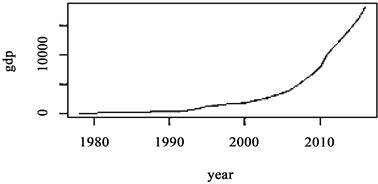Figure 1. Time series of Chongqing GDP from 1978 to 2022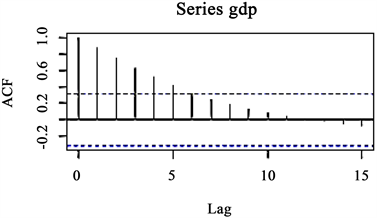Figure 2. ACF of Chongqing GDP

$\Delta {y}_{t}=\gamma {\gamma }_{t-1}+{\sum }_{i=1}^{p}{\beta }_{i}\Delta {y}_{t-i}+{\epsilon }_{i}$ (1)

$\Delta {y}_{t}=a+\gamma {\gamma }_{t-1}+{\sum }_{i=1}^{p}{\beta }_{i}\Delta {y}_{t-i}+{\epsilon }_{i}$ (2)

$\Delta {y}_{t}=a+\gamma {\gamma }_{t-1}+\delta t+{\sum }_{i=1}^{p}{\beta }_{i}\Delta {\gamma }_{t-i}+{\epsilon }_{i}$ (3)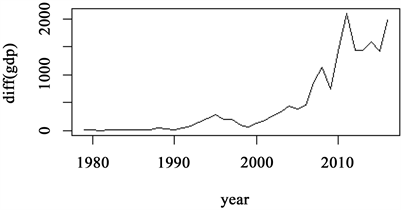Figure 3. Time series of the first differenced series of GDP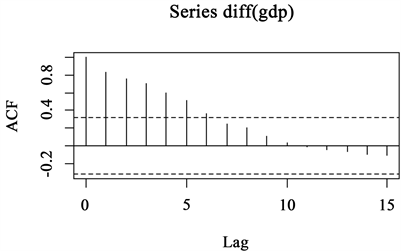Figure 4. ACF of the first differenced series of GDP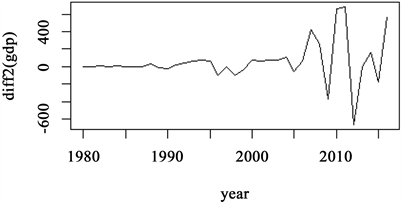Figure 5. Time series of the second differenced series of GDP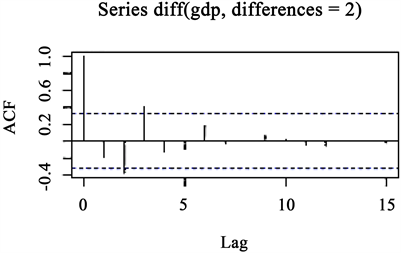Figure 6. ACF of the second differenced series of GDP

3.2. 确定ARIMA模型的阶数Figure 7. Results of EACF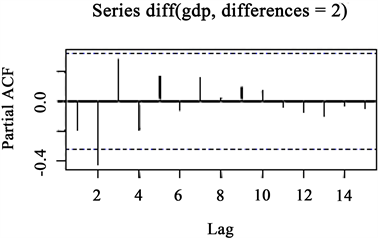Figure 8. PACF of the second differenced series of GDPTable 2. Comparison of different ARIMA models

3.3. 模型的检验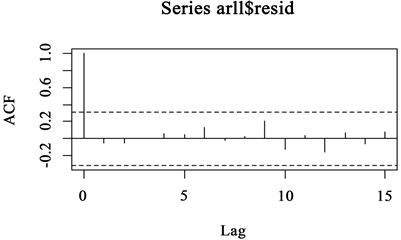Figure 9. ACF of residual series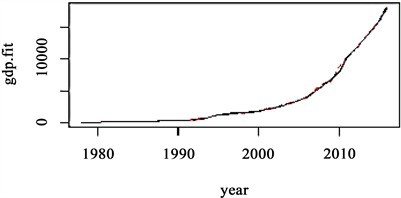Figure10. The fitted values (dashed line) and the actual observations (solid line) of GDP

3.4. 拟合和预测Table 3. Comparison of the forecasts and actual observations in ARIMA(2, 2, 2)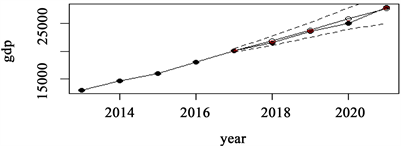Figure 11. The forecasts (hollow circles) and actual observations (solid circles), and dashed lines show 95% interval forecasts from 2017 to 2021

4. 结语

ARIMA模型只能做短期的预测，在实际应用过程中，ARIMA模型预测短期的效果很好，但预测时间的延长会导致模型的误差变大。另外，ARIMA模型仍是一种传统的线性时间序列模型，预测时仅仅考虑从历史看未来，而没有加入可能影响未来的各种因素，如重庆新冠肺炎疫情的严重性对其GDP的影响，还有“一带一路”对重庆经济的影响，这些都是未来影响GDP增长的因素，也是模型中所无法体现出来的。因此用ARIMA模型只以历史数据做短期的预测，仅以用来参考 。

  Tsay, R.S. (2010) Analysis of Financial Time Series Second Edition. John Wiley & Sons, Inc., Hoboken, New Jersey, 58-61. https://doi.org/10.1002/9780470644560  陈景祥. R软件应用统计方法[M]. 大连: 东北财经大学出版社, 2014: 558-572.  瞿海情, 何先平. 基于时间序列分析的湖北省GDP预测模型研究[J]. 湖北经济学院学报(人文社会科学版), 2021(9): 37-39.